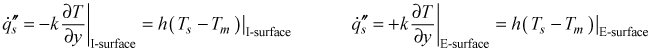# Institut für Thermodynamik der Luft- und Raumfahrt - Universität StuttgartMechanical Engineering - The University of Texas at Austin# heat transfer

Convective heat transfer occurs within a fluid wherever a wall temperature gradient exists. The most common way of establishing a temperature gradient within a wall-bounded internal flow is to create a temperature difference between the wall temperature and the bulk, mean, or mass-averaged temperature of the fluid that flows in the pipe or channel. Another way of creating temperature gradients within the fluid is from the effects of viscous dissipation, and this occurs most often with low-speed, high-Prandtl number flows, but it can also occur in a high-speed internal flow at any Prandtl number.

Sign Convention for Internal Flow Heat Transfer - TEXSTAN is programmed such that positive heat flux is into the fluid from both the I-surface and E-surface for all internal flows. For convenience Fourier's law for all internal geometries is transformed to use a common coordinate direction y, which extends out from a no-slip surface.

Surface Geometry - Internal flow geometries can be one of two general configurations: a symmetry line and a solid surface (pipe and symmetrical planar duct) or two solid surfaces (planar duct and annulus). For the pipe geometry (kgeom=4), where the radius r extends from the centerline (I-surface) to the wall (E-surface), the y-direction is from the wall towards the centerline. For the parallel planes channel geometry (kgeom=5,6), the y-direction extends from one no-slip surface (I-surface) to either the centerline (E-surface, for kgeom=5) or the second no-slip surface (E-surface, kgeom=6). For convenience we can choose kgeom=5 when there is the exact same thermal boundary condition on both surfaces. For the annulus geometry kgeom=7), the y-direction extends from one no-slip surface (I-surface at r=ri) to the other no-slip surface (E-surface at r=ro). The +y direction is summarized as follows:

• y = (rs - r)     pipe, referenced to E-surface (rs )
• y                  planar duct, referenced to I-surface
• y = (r - ri )     annulus, referenced to I-surface (ri )

Heat Transfer in Constant Property Flow - When constant properties are being considered, the stagnation enthalpy equation reduces to the temperature equation. For this flow the wall heat transfer is computed using the heat flux formulation of Fourier's law of heat conduction. The heat transfer coefficient is then defined by equating Fourier's law to Newton's law of cooling, based on the difference between the wall temperature and the mass-averaged fluid temperature. The mass-averaged temperature is the same as the mixed-mean temperature or the mean temperature or the bulk temperature. Technically the mass-averaged temperature is the temperature in the mass flow rate-specific heat-temperature triple product of the one-dimensional model of the First Law of Thermodynamics for flowing systems. The rate equations for conduction (Fourier's law) and convection (Newton's law of cooling) for each surface isNote that Fourier's law has a sign change at the E-surface to match the TEXSTAN requirement that positive heat flux is into the fluid (similar to what we often choose for the sign of heat in first-law thermodynamic ideas). For a pipe, a positive heat flux is into the fluid. For parallel-planes channel and an annulus, at the I-surface a positive heat flux is into the fluid and likewise at the E-surface a positive heat flux is also into the fluid. In all cases, a positive heat flux causes Tm(x) to increase with x. However, locally, at the I surface, a positive heat flux makes dT/dy>0 at the surface, and likewise, at the E-surface, a positive heat flux makes dT/dy>0 at that surface (because of the Fourier's Law sign change).

Finally, the heat transfer coefficient is recast into a nondimensional form using the traditional conduction-based Nusselt number for each wall:Heat Transfer in Variable Property Flow - The effects of variable properties on heat transfer in internal flows are generally due to one of two reasons: high speed flow (Mach number >0.4 causing significant viscous dissipation) or flows with large temperature differences. When variable properties are being considered, the thermal energy equation in TEXSTAN is the stagnation enthalpy equation. The formulations are similar to that described in the Heat Transfer in Variable Property Flow part of the external flows: heat transfer section of this website, with the free stream temperature being replaced by the mass-averaged temperature and with all properties in the nondimensional quantities are evaluated at the mass-averaged temperature. Viscous dissipation in internal flows can be considered with or without variable properties, but traditionally the adiabatic wall or recovery temperature is not adopted.

website updated Sept 2010   © 1996-2010 Michael E. Crawford - all rights reserved - website validated for CSS 2.1 and XHTML 1.0 strict at www.w3.org